# 7.3 Additional Properties of Ellipses

Let C be the ellipse with equation x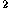/a+y/b=1, with a>b, and let F,F'=(±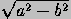,0) be its foci (see Figure 7.1.2 ).

• A parametric representation for C is given by

(a cos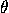, b sin).

The area of the shaded sector below is

½abab arccos(x/a).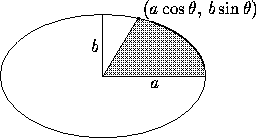The length of the arc from (a,0) to the point (a cos, b sin) is given by a( E(pi/2,e) - E(pi/2 - theta, e) ), where E is an elliptic integral (see the Standard Math Tables and Formulas for elliptic integrals). Setting=2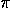we get

area C=ab,
perimeter C=4a E(pi/2,e).

• A rational parametric representation for C is given by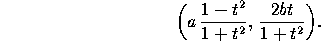• The polar equation for C in the usual polar coordinate system is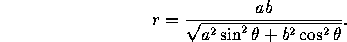With respect to a coordinate system with origin at a focus, the equation is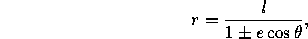where l=b/a is half the latus rectum. (Use the + sign for the focus with positive x-coordinate and the - sign for the other.)

• Let P be any point of C. The sum of the distances PF and PF' is constant, and equal to 2a.
• Let P be any point of C. Then the rays PF and PF' make the same angle with the tangent to C at P. Thus any light ray originating at F and reflected in the ellipse will also go through F'.
• Let T be any line tangent to C. The product of the distances from F and F' to T is constant, and equals b.
• Lahire's theorem: Let D and D' be fixed lines in the plane, and consider a third, moving, line on which three points P, P' and P'' are marked. If we constrain P to lie in D and P' to lie in D', then P'' describes an ellipse.

Next: 7.4 Additional Properties of Hyperbolas
Up: 7 Conics
Previous: 7.2 The General Quadratic EquationThe Geometry Center Home Page

Silvio Levy
Wed Oct 4 16:41:25 PDT 1995

This document is excerpted from the 30th Edition of the CRC Standard Mathematical Tables and Formulas (CRC Press). Unauthorized duplication is forbidden.